# Lorentz group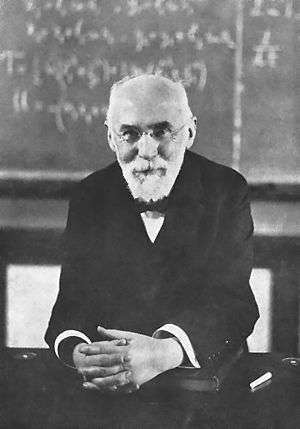Hendrik Antoon Lorentz (1853–1928), after whom the Lorentz group is named.

In physics and mathematics, the Lorentz group is the group of all Lorentz transformations of Minkowski spacetime, the classical and quantum setting for all (nongravitational) physical phenomena. The Lorentz group is named for the Dutch physicist Hendrik Lorentz.

For example, the following laws, equations, and theories respect Lorentz symmetry:

The Lorentz group expresses the fundamental symmetry of space and time of all known fundamental laws of nature. In general relativity physics is that of special relativity in small enough regions of spacetime.

## Basic properties

The Lorentz group is a subgroup of the Poincaré group—the group of all isometries of Minkowski spacetime. Lorentz transformations are, precisely, isometries that leave the origin fixed. Thus, the Lorentz group is an isotropy subgroup of the isometry group of Minkowski spacetime. For this reason, the Lorentz group is sometimes called the homogeneous Lorentz group while the Poincaré group is sometimes called the inhomogeneous Lorentz group. Lorentz transformations are examples of linear transformations; general isometries of Minkowski spacetime are affine transformations. Mathematically, the Lorentz group may be described as the generalized orthogonal group O(1,3), the matrix Lie group that preserves the quadratic formon R4. This quadratic form is, when put on matrix form (see classical orthogonal group), interpreted in physics as the metric tensor of Minkowski spacetime.

The Lorentz group is a six-dimensional noncompact non-abelian real Lie group that is not connected. The four connected components are not simply connected, but rather doubly connected. The identity component (i.e., the component containing the identity element) of the Lorentz group is itself a group, and is often called the restricted Lorentz group, and is denoted SO+(1,3). The restricted Lorentz group consists of those Lorentz transformations that preserve the orientation of space and direction of time. The restricted Lorentz group has often been presented through a facility of biquaternion algebra.

The restricted Lorentz group arises in other ways in pure mathematics. For example, it arises as the point symmetry group of a certain ordinary differential equation. This fact also has physical significance.

### Connected components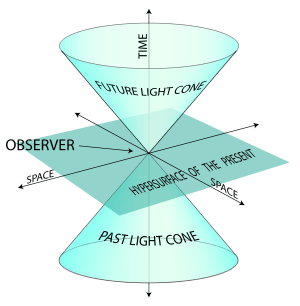Light cone in 2D space plus a time dimension.

Because it is a Lie group, the Lorentz group O(1,3) is both a group and admits a topological description as a smooth manifold. As a manifold, it has four connected components. Intuitively, this means that it consists of four topologically separated pieces.

The four connected components can be categorized by two transformation properties its elements have:

• some elements are reversed under time-inverting Lorentz transformations, for example, a future-pointing timelike vector would be inverted to a past-pointing vector
• some elements have orientation reversed by improper Lorentz transformations, for example, certain vierbein (tetrads)

Lorentz transformations that preserve the direction of time are called orthochronous. The subgroup of orthochronous transformations is often denoted O+(1,3). Those that preserve orientation are called proper, and as linear transformations they have determinant +1. (The improper Lorentz transformations have determinant −1.) The subgroup of proper Lorentz transformations is denoted SO(1,3).

The subgroup of all Lorentz transformations preserving both orientation and direction of time is called the proper, orthochronous Lorentz group or restricted Lorentz group, and is denoted by SO+(1, 3). (Note that some authors refer to SO(1,3) or even O(1,3) when they actually mean SO+(1, 3).)

The set of the four connected components can be given a group structure as the quotient group O(1,3)/SO+(1,3), which is isomorphic to the Klein four-group. Every element in O(1,3) can be written as the semidirect product of a proper, orthochronous transformation and an element of the discrete group

{1, P, T, PT}

where P and T are the space inversion and time reversal operators:

P = diag(1, −1, −1, −1)
T = diag(−1, 1, 1, 1).

Thus an arbitrary Lorentz transformation can be specified as a proper, orthochronous Lorentz transformation along with a further two bits of information, which pick out one of the four connected components. This pattern is typical of finite-dimensional Lie groups.

## Restricted Lorentz group

The restricted Lorentz group is the identity component of the Lorentz group, which means that it consists of all Lorentz transformations that can be connected to the identity by a continuous curve lying in the group. The restricted Lorentz group is a connected normal subgroup of the full Lorentz group with the same dimension, in this case with dimension six.

The restricted Lorentz group is generated by ordinary spatial rotations and Lorentz boosts (which can be thought of as hyperbolic rotations in a plane that includes a time-like direction). Since every proper, orthochronous Lorentz transformation can be written as a product of a rotation (specified by 3 real parameters) and a boost (also specified by 3 real parameters), it takes 6 real parameters to specify an arbitrary proper orthochronous Lorentz transformation. This is one way to understand why the restricted Lorentz group is six-dimensional. (See also the Lie algebra of the Lorentz group.)

The set of all rotations forms a Lie subgroup isomorphic to the ordinary rotation group SO(3). The set of all boosts, however, does not form a subgroup, since composing two boosts does not, in general, result in another boost. (Rather, a pair of non-colinear boosts is equivalent to a boost and a rotation, and this relates to Thomas rotation.) A boost in some direction, or a rotation about some axis, generates a one-parameter subgroup.

### Surfaces of transitivity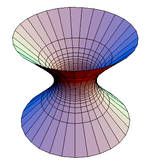Hyperboloid of one sheet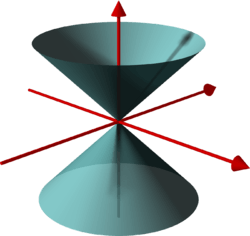Common conical surface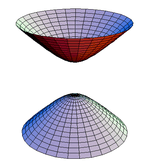Hyperboloid of two sheets

If a group G acts on a space V, then a surface SV is a surface of transitivity if S is invariant under G, i.e., gsSgG, ∀sS, and for any two points s1, s2S there is a gG such that gs1 = s2. By definition of the Lorentz group, it preserves the quadratic formThe surfaces of transitivity of the orthochronous Lorentz group O+(1, 3), Q(x) = const. of spacetime are the following:

• Q(x) > 0, x0 > 0 is the upper branch of a hyperboloid of two sheets.
• Q(x) > 0, x0 < 0 is the lower branch of this hyperboloid.
• Q(x) = 0, x0 > 0 is the upper branch of the light cone.
• Q(x) = 0, x0 < 0 is the lower branch of the light cone.
• Q(x) < 0 is a hyperboloid of one sheet.
• The origin x0 = x1 = x2 = x3 = 0.

These surfaces are 3-dimensional, so the images are not faithful, but they are faithful for the corresponding facts about O+(1, 2). For the full Lorentz group, the surfaces of transitivity are only four since the transformation T takes an upper branch of a hyperboloid (cone) to a lower one and vice versa.

These observations constitute a good starting point for finding all infinite-dimensional unitary representations of the Lorentz group, in fact, of the Poincaré group, using the method of induced representations. One begins with a "standard vector", one for each surface of transitivity, and then ask which subgroup preserves these vectors. These subgroups are called little groups by physicists. The problem is then essentially reduced to the easier problem of finding representations of the little groups. For example, a standard vector in one of the hyperbolas of two sheets could be suitably chosen as (m, 0, 0, 0). For each m ≠ 0, the vector pierces exactly one sheet. In this case the little group is SO(3), the rotation group, all of whose representations are known. The precise infinite-dimensional unitary representation under which a particle transforms is part of its classification. Not all representations can correspond to physical particles (as far as is known). Standard vectors on the one-sheeted hyperbolas would correspond to tachyons. Particles on the light cone are photons, and more hypothetically, gravitons. The "particle" corresponding to the origin is the vacuum.

### Relation to the Möbius group

The restricted Lorentz group SO+(1, 3) is isomorphic to the projective special linear group PSL(2,C), which is in turn isomorphic to the Möbius group, the symmetry group of conformal geometry on the Riemann sphere. (This observation was utilized by Roger Penrose as the starting point of twistor theory.)

This may be shown by constructing a surjective homomorphism of Lie groups from SL(2,C) to SO+(1,3), which we will call the spinor map. This proceeds as follows:

We can define an action of SL(2,C) on Minkowski spacetime by writing a point of spacetime as a two-by-two Hermitian matrix in the formThis presentation has the pleasant feature thatTherefore, we have identified the space of Hermitian matrices (which is four-dimensional, as a real vector space) with Minkowski spacetime in such a way that the determinant of a Hermitian matrix is the squared length of the corresponding vector in Minkowski spacetime. SL(2,C) acts on the space of Hermitian matrices viawhereis the Hermitian transpose of, and this action preserves the determinant. Therefore, SL(2,C) acts on Minkowski spacetime by (linear) isometries. This defines a map from SL(2,C) to the Lorentz group SO+(1,3), and the map is evidently a homomorphism. This is the spinor map.

The kernel of the spinor map is the two element subgroup ±I, and it happens that the map is surjective. By the first isomorphism theorem, the quotient group PSL(2,C) = SL(2,C) / {±I} is isomorphic to SO+(1,3).

#### Appearance of the night sky

This isomorphism has the consequence that Möbius transformations of the Riemann sphere represent the way that Lorentz transformations change the appearance of the night sky, as seen by an observer who is maneuvering at relativistic velocities relative to the "fixed stars".

Suppose the "fixed stars" live in Minkowski spacetime and are modeled by points on the celestial sphere. Then a given point on the celestial sphere can be associated with ξ = u + iv, a complex number that corresponds to the point on the Riemann sphere, and can be identified with a null vector (a light-like vector) in Minkowski spaceor the Hermitian matrixThe set of real scalar multiples of this null vector, called a null line through the origin, represents a line of sight from an observer at a particular place and time (an arbitrary event we can identify with the origin of Minkowski spacetime) to various distant objects, such as stars. Then the points of the celestial sphere (equivalently, lines of sight) are identified with certain Hermitian matrices.

### Conjugacy classes

Because the restricted Lorentz group SO+(1, 3) is isomorphic to the Möbius group PSL(2,C), its conjugacy classes also fall into five classes:

• Elliptic transformations
• Hyperbolic transformations
• Loxodromic transformations
• Parabolic transformations
• The trivial identity transformation

In the article on Möbius transformations, it is explained how this classification arises by considering the fixed points of Möbius transformations in their action on the Riemann sphere, which corresponds here to null eigenspaces of restricted Lorentz transformations in their action on Minkowski spacetime.

An example of each type is given in the subsections below, along with the effect of the one-parameter subgroup it generates (e.g., on the appearance of the night sky).

The Möbius transformations are the conformal transformations of the Riemann sphere (or celestial sphere). Then conjugating with an arbitrary element of SL(2,C) obtains the following examples of arbitrary elliptic, hyperbolic, loxodromic, and parabolic (restricted) Lorentz transformations, respectively. The effect on the flow lines of the corresponding one-parameter subgroups is to transform the pattern seen in the examples by some conformal transformation. For example, an elliptic Lorentz transformation can have any two distinct fixed points on the celestial sphere, but points still flow along circular arcs from one fixed point toward the other. The other cases are similar.

#### Elliptic

An elliptic element of SL(2,C) isand has fixed points ξ = 0, ∞. Writing the action as XP1 X P1* and collecting terms, the spinor map converts this to the (restricted) Lorentz transformationThis transformation then represents a rotation about the z axis, exp(iθJz). The one-parameter subgroup it generates is obtained by taking θ to be a real variable, the rotation angle, instead of a constant.

The corresponding continuous transformations of the celestial sphere (except for the identity) all share the same two fixed points, the North and South poles. The transformations move all other points around latitude circles so that this group yields a continuous counterclockwise rotation about the z axis as θ increases. The angle doubling evident in the spinor map is a characteristic feature of spinorial double coverings.

#### Hyperbolic

A hyperbolic element of SL(2,C) isand has fixed points ξ = 0, ∞. Under stereographic projection from the Riemann sphere to the Euclidean plane, the effect of this Möbius transformation is a dilation from the origin.

The spinor map converts this to the Lorentz transformationThis transformation represents a boost along the z axis with rapidity β. The one-parameter subgroup it generates is obtained by taking β to be a real variable, instead of a constant. The corresponding continuous transformations of the celestial sphere (except for the identity) all share the same fixed points (the North and South poles), and they move all other points along longitudes away from the South pole and toward the North pole.

#### Loxodromic

A loxodromic element of SL(2,C) isand has fixed points ξ = 0, ∞. The spinor map converts this to the Lorentz transformationThe one-parameter subgroup this generates is obtained by replacing β+iθ with any real multiple of this complex constant. (If β, θ vary independently, then a two-dimensional abelian subgroup is obtained, consisting of simultaneous rotations about the z axis and boosts along the z-axis; in contrast, the one-dimensional subgroup discussed here consists of those elements of this two-dimensional subgroup such that the rapidity of the boost and angle of the rotation have a fixed ratio.)

The corresponding continuous transformations of the celestial sphere (excepting the identity) all share the same two fixed points (the North and South poles). They move all other points away from the South pole and toward the North pole (or vice versa), along a family of curves called loxodromes. Each loxodrome spirals infinitely often around each pole.

#### Parabolic

A parabolic element of SL(2,C) isand has the single fixed point ξ = ∞ on the Riemann sphere. Under stereographic projection, it appears as an ordinary translation along the real axis.

The spinor map converts this to the matrix (representing a Lorentz transformation)This generates a two-parameter abelian subgroup, which is obtained by considering α a complex variable rather than a constant. The corresponding continuous transformations of the celestial sphere (except for the identity transformation) move points along a family of circles that are all tangent at the North pole to a certain great circle. All points other than the North pole itself move along these circles.

Parabolic Lorentz transformations are often called null rotations, since they preserve null vectors, just as rotations preserve timelike vectors and boosts preserve spacelike vectors. Since these are likely to be the least familiar of the four types of nonidentity Lorentz transformations (elliptic, hyperbolic, loxodromic, parabolic), it is illustrated here how to determine the effect of an example of a parabolic Lorentz transformation on Minkowski spacetime.

The matrix given above yields the transformationNow, without loss of generality, pick Im(α)=0. Differentiating this transformation with respect to the now real group parameter α and evaluating at α=0 produces the corresponding vector field (first order linear partial differential operator),Apply this to a function f(t,x,y,z), and demand that it stays invariant, i.e., it is annihilated by this transformation. The solution of the resulting first order linear partial differential equation can be expressed in the formwhere F is an arbitrary smooth function. The arguments of F give three rational invariants describing how points (events) move under this parabolic transformation, as they themselves do not move,Choosing real values for the constants on the right hand sides yields three conditions, and thus specifies a curve in Minkowski spacetime. This curve is an orbit of the transformation.

The form of the rational invariants shows that these flowlines (orbits) have a simple description: suppressing the inessential coordinate y, each orbit is the intersection of a null plane, t = z+c2, with a hyperboloid, t2−x2−z2 = c3. The case c3 = 0 has the hyperboloid degenerate to a light cone with the orbits becoming parabolas lying in corresponding null planes.

A particular null line lying on the light cone is left invariant; this corresponds to the unique (double) fixed point on the Riemann sphere mentioned above. The other null lines through the origin are "swung around the cone" by the transformation. Following the motion of one such null line as α increases corresponds to following the motion of a point along one of the circular flow lines on the celestial sphere, as described above.

A choice Re(α)=0 instead, produces similar orbits, now with the roles of x and y interchanged.

Parabolic transformations lead to the gauge symmetry of massless particles (like photons) with helicity |h| ≥ 1. In the above explicit example, a massless particle moving in the z direction, so with 4-momentum P=(p,0,0,p), is not affected at all by the x-boost and y-rotation combination Kx−Jy displayed above, in the "little group" of its motion. This is evident from the explicit transformation law discussed: like any light-like vector, P itself is now invariant, i.e., all traces or effects of α have disappeared. c1 = c2 = c3 = 0, in the special case discussed. (The other similar generator, Ky+Jx as well as it and Jz comprise altogether the little group of the lightlike vector, isomorphic to E(2).)

## Lie algebra

As with any Lie group, the best way to study many aspects of the Lorentz group is via its Lie algebra. The Lorentz group is a subgroup of the diffeomorphism group of R4 and therefore its Lie algebra can be identified with vector fields on R4. In particular, the vectors that generate isometries on a space are its Killing vectors, which provides a convenient alternative to the left-invariant vector field for calculating the Lie algebra. We can write down a set of six generators:

• vector fields on R4 generating three rotations i J,• vector fields on R4 generating three boosts i K,It may be helpful to briefly recall here how to obtain a one-parameter group from a vector field, written in the form of a first order linear partial differential operator such asThe corresponding initial value problem isThe solution can be writtenorwhere we easily recognize the one-parameter matrix group of rotations exp(i λ Jz) about the z axis. Differentiating with respect to the group parameter λ and setting it λ=0 in that result, we recover the standard matrix,which corresponds to the vector field we started with. This illustrates how to pass between matrix and vector field representations of elements of the Lie algebra.

Reversing the procedure in the previous section, we see that the Möbius transformations that correspond to our six generators arise from exponentiating respectively β/2 (for the three boosts) or /2 (for the three rotations) times the three Pauli matricesFor our purposes, another generating set is more convenient. The following table lists the six generators, in which

• The first column gives a generator of the flow under the Möbius action (after stereographic projection from the Riemann sphere) as a real vector field on the Euclidean plane.
• The second column gives the corresponding one-parameter subgroup of Möbius transformations.
• The third column gives the corresponding one-parameter subgroup of Lorentz transformations (the image under our homomorphism of preceding one-parameter subgroup).
• The fourth column gives the corresponding generator of the flow under the Lorentz action as a real vector field on Minkowski spacetime.

Notice that the generators consist of

• Two parabolics (null rotations)
• One hyperbolic (boost in the ∂z direction)
• Three elliptics (rotations about the x,y,z axes, respectively)
Vector field on R2 One-parameter subgroup of SL(2,C),
representing Möbius transformations
One-parameter subgroup of SO+(1,3),
representing Lorentz transformations
Vector field on R4
ParabolicHyperbolicEllipticExponentiate:This element of SL(2,C) represents the one-parameter subgroup of (elliptic) Möbius transformations:Next,The corresponding vector field on C (thought of as the image of S2 under stereographic projection) isWriting, this becomes the vector field on R2Returning to our element of SL(2,C), writing out the actionand collecting terms, we find that the image under the spinor map is the element of SO+(1,3)Differentiating with respect to θ at θ=0, yields the corresponding vector field on R4,This is evidently the generator of counterclockwise rotation about the y axis.

## Subgroups of the Lorentz group

The subalgebras of the Lie algebra of the Lorentz group can be enumerated, up to conjugacy, from which we can list the closed subgroups of the restricted Lorentz group, up to conjugacy. (See the book by Hall cited below for the details.) We can readily express the result in terms of the generating set given in the table above.

The one-dimensional subalgebras of course correspond to the four conjugacy classes of elements of the Lorentz group:

•generates a one-parameter subalgebra of parabolics SO(0,1),
•generates a one-parameter subalgebra of boosts SO(1,1),
•generates a one-parameter of rotations SO(2),
•(for any) generates a one-parameter subalgebra of loxodromic transformations.

(Strictly speaking the last corresponds to infinitely many classes, since distinctgive different classes.) The two-dimensional subalgebras are:

•generate an abelian subalgebra consisting entirely of parabolics,
•generate a nonabelian subalgebra isomorphic to the Lie algebra of the affine group A(1),
•generate an abelian subalgebra consisting of boosts, rotations, and loxodromics all sharing the same pair of fixed points.

The three-dimensional subalgebras are:

•generate a Bianchi V subalgebra, isomorphic to the Lie algebra of Hom(2), the group of euclidean homotheties,
•generate a Bianchi VII_0 subalgebra, isomorphic to the Lie algebra of E(2), the euclidean group,
•, where, generate a Bianchi VII_a subalgebra,
•generate a Bianchi VIII subalgebra, isomorphic to the Lie algebra of SL(2,R), the group of isometries of the hyperbolic plane,
•generate a Bianchi IX subalgebra, isomorphic to the Lie algebra of SO(3), the rotation group.

(Here, the Bianchi types refer to the classification of three-dimensional Lie algebras by the Italian mathematician Luigi Bianchi.) The four-dimensional subalgebras are all conjugate to

•generate a subalgebra isomorphic to the Lie algebra of Sim(2), the group of Euclidean similitudes.

The subalgebras form a lattice (see the figure), and each subalgebra generates by exponentiation a closed subgroup of the restricted Lie group. From these, all subgroups of the Lorentz group can be constructed, up to conjugation, by multiplying by one of the elements of the Klein four-group.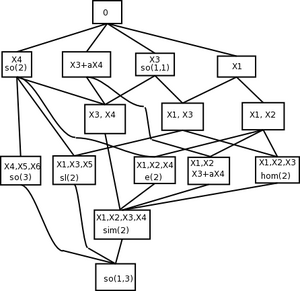The lattice of subalgebras of the Lie algebra SO(1,3), up to conjugacy.

As with any connected Lie group, the coset spaces of the closed subgroups of the restricted Lorentz group, or homogeneous spaces, have considerable mathematical interest. A few, brief descriptions:

• The group Sim(2) is the stabilizer of a null line, i.e., of a point on the Riemann sphere—so the homogeneous space SO+(1,3)/Sim(2) is the Kleinian geometry that represents conformal geometry on the sphere S.2
• The (identity component of the) Euclidean group SE(2) is the stabilizer of a null vector, so the homogeneous space SO+(1,3)/SE(2) is the momentum space of a massless particle; geometrically, this Kleinian geometry represents the degenerate geometry of the light cone in Minkowski spacetime.
• The rotation group SO(3) is the stabilizer of a timelike vector, so the homogeneous space SO+(1,3)/SO(3) is the momentum space of a massive particle; geometrically, this space is none other than three-dimensional hyperbolic space H3.

## Covering groups

In a previous section, we constructed a homomorphism SL(2, C) → SO+(1, 3), which we called the spinor map. Since SL(2,C) is simply connected, it is the covering group of the restricted Lorentz group SO+(1, 3). By restriction we obtain a homomorphism SU(2) → SO(3). Here, the special unitary group SU(2), which is isomorphic to the group of unit norm quaternions, is also simply connected, so it is the covering group of the rotation group SO(3). Each of these covering maps are twofold covers in the sense that precisely two elements of the covering group map to each element of the quotient. One often says that the restricted Lorentz group and the rotation group are doubly connected. This means that the fundamental group of the each group is isomorphic to the two-element cyclic group Z2.

(In applications to quantum mechanics, the special linear group SL(2, C) is sometimes called the Lorentz group.)

Twofold coverings are characteristic of spin groups. Indeed, in addition to the double coverings

Spin+(1, 3) = SL(2, C) → SO+(1, 3)
Spin(3) = SU(2) → SO(3)

we have the double coverings

Pin(1, 3) → O(1, 3)
Spin(1, 3) → SO(1, 3)
Spin+(1, 2) = SU(1, 1) → SO(1, 2)

These spinorial double coverings are all closely related to Clifford algebras.

## Topology

The left and right groups in the double covering

SU(2) → SO(3)

are deformation retracts of the left and right groups, respectively, in the double covering

SL(2,C) → SO+(1,3).

But the homogeneous space SO+(1,3)/SO(3) is homeomorphic to hyperbolic 3-space H3, so we have exhibited the restricted Lorentz group as a principal fiber bundle with fibers SO(3) and base H3. Since the latter is homeomorphic to R3, while SO(3) is homeomorphic to three-dimensional real projective space RP3, we see that the restricted Lorentz group is locally homeomorphic to the product of RP3 with R3. Since the base space is contractible, this can be extended to a global homeomorphism.

## Generalization to higher dimensions

The concept of the Lorentz group has a natural generalization to spacetime of any number of dimensions. Mathematically, the Lorentz group of n+1-dimensional Minkowski space is the group O(n,1) (or O(1,n)) of linear transformations of Rn+1 that preserves the quadratic formMany of the properties of the Lorentz group in four dimensions (where n = 3) generalize straightforwardly to arbitrary n. For instance, the Lorentz group O(n,1) has four connected components, and it acts by conformal transformations on the celestial (n−1)-sphere in n+1-dimensional Minkowski space. The identity component SO+(n,1) is an SO(n)-bundle over hyperbolic n-space Hn.

The low-dimensional cases n = 1 and n = 2 are often useful as "toy models" for the physical case n = 3, while higher-dimensional Lorentz groups are used in physical theories such as string theory that posit the existence of hidden dimensions. The Lorentz group O(n,1) is also the isometry group of n-dimensional de Sitter space dSn, which may be realized as the homogeneous space O(n,1)/O(n−1,1). In particular O(4,1) is the isometry group of the de Sitter universe dS4, a cosmological model.

## References

• Artin, Emil (1957). Geometric Algebra. New York: Wiley. ISBN 0-471-60839-4. See Chapter III for the orthogonal groups O(p,q).
• Carmeli, Moshe (1977). Group Theory and General Relativity, Representations of the Lorentz Group and Their Applications to the Gravitational Field. McGraw-Hill, New York. ISBN 0-07-009986-3. A canonical reference; see chapters 1–6 for representations of the Lorentz group.
• Frankel, Theodore (2004). The Geometry of Physics (2nd Ed.). Cambridge: Cambridge University Press. ISBN 0-521-53927-7. An excellent resource for Lie theory, fiber bundles, spinorial coverings, and many other topics.
• Fulton, William; Harris, Joe (1991). Representation theory. A first course. Graduate Texts in Mathematics, Readings in Mathematics. 129. New York: Springer-Verlag. ISBN 978-0-387-97495-8. MR 1153249, ISBN 978-0-387-97527-6. See Lecture 11 for the irreducible representations of SL(2,C).
• Gelfand, I.M.; Minlos, R.A.; Shapiro, Z.Ya. (1963), Representations of the Rotation and Lorentz Groups and their Applications, New York: Pergamon Press
• Hall, G. S. (2004). Symmetries and Curvature Structure in General Relativity. Singapore: World Scientific. ISBN 981-02-1051-5. See Chapter 6 for the subalgebras of the Lie algebra of the Lorentz group.
• Hatcher, Allen (2002). Algebraic topology. Cambridge: Cambridge University Press. ISBN 0-521-79540-0. See also the "online version". Retrieved July 3, 2005. See Section 1.3 for a beautifully illustrated discussion of covering spaces. See Section 3D for the topology of rotation groups.
• Naber, Gregory (1992). The Geometry of Minkowski Spacetime. New York: Springer-Verlag. ISBN 0486432351. (Dover reprint edition.) An excellent reference on Minkowski spacetime and the Lorentz group.
• Needham, Tristan (1997). Visual Complex Analysis. Oxford: Oxford University Press. ISBN 0-19-853446-9. See Chapter 3 for a superbly illustrated discussion of Möbius transformations.
• Weinberg, S. (2002), The Quantum Theory of Fields, 1, Cambridge University Press, ISBN 0-521-55001-7
• Wigner, E. P. (1939), "On unitary representations of the inhomogeneous Lorentz group", Annals of Mathematics, 40 (1): 149–204, Bibcode:1939AnMat..40..922E, doi:10.2307/1968551, MR 1503456.